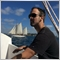# Set a delay timer between trades138

Im wondering if its possible to set a delay timer between trades? I'm working on automating a strategy, but there are some times when conditions are met that trades will open and close faster then you can blink. I'm wondering if we can say "For the next 10 bars, do not open any new trades", or "For the next 60 minutes do not open any new trades"

Would really like someone's assistance if possible... thx3196

first u can use sleep()

second u can use timing for example (something like this)

```int WaitTime = 10;  // 10 Min.
static int TimeSent;
if (TimeCurrent() >= TimeSent + (WaitTime * 60))
{
OrderSend(.....);
TimeSent = TimeCurrent();
}
else
return(0);
```

or u can use bars option for example (something like this)

```int WaitBars = 10;  // 10 Bars.
static int TimeSent;
int shift = iBarShift(NULL,0,TimeSent);
if (shift >= WaitBars)
{
OrderSend(.....);
TimeSent = Time;
}
else
return(0);
```

didn't checked

& there r a lot more options138

qjol:

first u can use sleep()

second u can use timing for example (something like this)

or u can use bars option for example (something like this)

didn't checked

& there r a lot more options

I love you. Thx ;)[Deleted]

Ok...a new thought to add to this ...if you've got time it would be much appreciated.

> How would I pause trading after one bad trade for a period of say 1 'current' bars or 24hours for instance?

Thanks,

DAVID17725

Wooopa:

Ok...a new thought to add to this ...if you've got time it would be much appreciated.

> How would I pause trading after one bad trade for a period of say 1 'current' bars or 24hours for instance?

Thanks,

DAVID

Please don't double post . . .12

I have add the same code to my EA (very simple only for testing) but I am missing something please can someone guide me.

I am getting the error  'return' - 'void' function returns a value.

Thankyou so much

```#include<Trade\Trade.mqh>
#include <Math\Stat\Normal.mqh>
#include <Expert\Expert.mqh>

CPositionInfo  m_position;                   // trade position object
COrderInfo     m_order;                      // trade order object
CSymbolInfo    m_symbol;

void OnTick()
{

//-----------------------------------------------------------------------------------------

// get the ask price

int  NumberOfPositions=0; // number of positions

//+------------------------------------------------------------------+
//|   Information for the Buy Order                                  |
//+------------------------------------------------------------------+

int WaitTime = 10;  // 10 Min.
static int TimeSent;
if (TimeCurrent() >= TimeSent + (WaitTime * 60));

if (NumberOfPositions<10)

{
TimeSent = TimeCurrent();
}
else
return(0);

//+------------------------------------------------------------------+
//| Sell if Ticket has Profit                                      |
//+------------------------------------------------------------------+
{

for(int i=PositionsTotal()-1;i>=0;i--) // returns the number of current positions
if(m_position.SelectByIndex(i))     // selects the position by index for further access to its properties
if( m_position.Symbol() == Symbol())
if(m_position.Profit()>0.60)
m_trade.PositionClose(m_position.Ticket()); // close a position by the specified symbol

}

}
```5285

micke81:

I have add the same code to my EA (very simple only for testing) but I am missing something please can someone guide me.

I am getting the error  'return' - 'void' function returns a value.

Thankyou so much

```#include<Trade\Trade.mqh>
#include <Math\Stat\Normal.mqh>
#include <Expert\Expert.mqh>

CPositionInfo  m_position;                   // trade position object
COrderInfo     m_order;                      // trade order object
CSymbolInfo    m_symbol;

void OnTick()
{

//-----------------------------------------------------------------------------------------

// get the ask price

int  NumberOfPositions=0; // number of positions

//+------------------------------------------------------------------+
//|   Information for the Buy Order                                  |
//+------------------------------------------------------------------+

int WaitTime = 10;  // 10 Min.
static int TimeSent;
if (TimeCurrent() >= TimeSent + (WaitTime * 60));

if (NumberOfPositions<10)

{
TimeSent = TimeCurrent();
}
else
return(0);//replace with only return;

//+------------------------------------------------------------------+
//| Sell if Ticket has Profit                                      |
//+------------------------------------------------------------------+
{

for(int i=PositionsTotal()-1;i>=0;i--) // returns the number of current positions
if(m_position.SelectByIndex(i))     // selects the position by index for further access to its properties
if( m_position.Symbol() == Symbol())
if(m_position.Profit()>0.60)
m_trade.PositionClose(m_position.Ticket()); // close a position by the specified symbol

}

}```12

Kenneth Parling: